Short articles on topics in systematic trading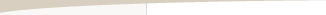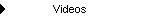Other Articles...

Significance testingSignificance Testing

by Michael R. Bryant

A statistical significance test can be used to help determine if a trading system or method is likely to be profitable in the future. Specifically, a Student's t test can be applied to the average trade for the trading system or method under consideration. The test determines if the average trade is significantly greater than zero at a specified confidence level. For example, the test will determine if the average trade is greater than zero with, say, 95% confidence.

The test requires the number of rules and/or restrictions imposed by the trading system or method. The number of rules and/or restrictions is used to calculate the number of degrees of freedom, which is necessary to calculate the t value for the t test. There needs to be a sufficient number of degrees of freedom to insure that the system is not over-fit or over-optimized to the market. Over-fitting or over-optimization means that the trading system's parameters have been selected to work on specific markets or under limited market conditions. An over-fit or over-optimized trading system is unlikely to perform well on other markets or when market conditions change. Most trading experts agree that over-optimized systems should be avoided.

The number of degrees of freedom is the number of trades minus the number of restrictions. With too few trades, the profitability of the system or method may be due to a chance arrangement of trades. The more trades, the greater the number of degrees of freedom and the more likely it is that the calculated average profit is not a statistical fluke but a real number that is likely to hold up in the future.

To count the number of restrictions, Thomas Hoffman (Babcock, Bruce. The Business One Irwin Guide to Trading Systems. Richard D. Irwin, Inc. 1989, p. 89) suggests examining a trading system's rules and counting any condition that would change the resulting trades. For example, suppose you have a trading system that buys when today's close is less than yesterday's close in an up trend. It defines an up trend as when a shorter moving average is greater than a longer moving average. For simplicity, assume the sell side is the reverse, and there are no stops. It's a simple stop and reverse system.

The moving average cross-over condition would probably be counted as three restrictions: one for the condition itself, and one for each moving average period. The price pattern would be another restriction for a total of four restrictions for the long side. There would be four more for the short side for a total of eight restrictions. If there were only eight trades, for example, there would be no degrees of freedom, and you shouldn't have any confidence in the average trade number, even if it were very high. On the other hand, if there were 100 trades, there would be 92 degrees of freedom, which should give you much more confidence in the average trade number.

The t test can be expressed as a confidence interval for the average trade:

CI = t * SD/sqrt(N)

where CI is the confidence interval around the average trade, t is the Student's t statistic, SD is the standard deviation of the trades, N is the number of trades, and sqrt represents "square root." The t statistic depends on the number of degrees of freedom and the confidence level.

The confidence interval means that the average trade is likely to lie between T - CI and T + CI. For the system to be profitable at the specified confidence level, the average trade, T, needs to be greater than zero at the lower bound, T - CI; i.e.,

T > CI.

If this condition is true at the specified confidence level, it means that the system or method is inherently profitable subject to the assumptions of the test. One of these assumptions is that the statistical properties of the trades remain the same. Specifically, if the average trade and its standard deviation remain the same in the future, the results will continue to be valid. However, as markets change and evolve over time, the properties of the statistical distribution of trades may change as well, so caution is warranted in interpreting the results.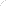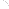Join Our Email List Email: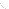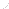For Email Marketing you can trust